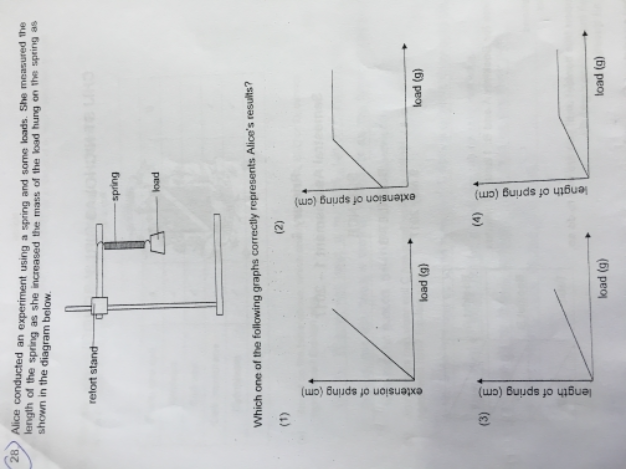# QuestionHi,

Is the correct answer (1)? Pls help.

Thanks

I think the answer is (2).

Answers (3) and (4) are out because the length of the spring (vertical axis) cannot start from zero – since the spring has a length.

The question also stated she measured the experiment as she increased the load => there was already a mass on the spring => that the extension is not zero, therefore the answer is not 1).

As she increased the mass, the extension increased to a maximum (the spring was fully stretched and could no longer be extended) and then the extension of the spring remained constant after that even as the load increased.

1 Reply 0 Likes

Wah.. like that it becomes a word game, rather than a test of science concept. Actually my kid answered (2), same reasoning as yours. But answer sheet is (1).

I thought that (1) can be correct too if the mass added has not reached the maximum point where the spring lose its elasticity. But of course if at the start of the experiment there was already a load, (1) is wrong. The question is not very clear on this fact.

1 Reply 0 Likes

Actually I agree with you. I also thought that (1) is correct if the mass has not reached the point at which the spring is fully stretched.

I’m assuming, though, that they are trying to see if the kids get the concept of there being a maximum length of extension…. but yes, there’s an element of second guessing here.

0 Replies 0 Likes